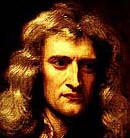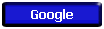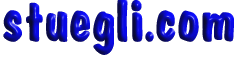#Newton's Laws

 1st "Every object continues in its state of rest , or of motion in a straight line at a constant speed, unless it is compelled to change that state by forces exerted upon it" 2nd "The acceleration produced by a net force on an object is directly proportional to the magnitude of the net force, is in the same direction as the net force, and is inversely proportional to the mass of the object." 3rd "Whenever an object exerts a force on a second object, the second object exerts an equal and opposite force on the first."

# Sir Issac Newton Publishes His Animation Principles Of Motion

Issac Newton, English physicist and mathematician was born into a poor farming family. Luckily for humanity, Newton was not a good farmer, and was sent to Cambridge to study to become a preacher. At Cambridge, Newton studied mathematics, being especially strongly influenced by Euclid, although he was also influenced by Baconian and Cartesian philosophies. Newton was forced to leave Cambridge when it was closed because of the plague, and it was during this period that he made some of his most significant discoveries.
Newton suffered a mental breakdown in 1675 and was still recovering through 1679. In response to a letter from Hooke, he suggested that a particle, if released, would spiral in to the center of the Earth. Hooke wrote back, claiming that the path would not be a spiral, but an ellipse. Newton, who hated being bested, then proceeded to work out the mathematics of orbits. Newton then began devoting his efforts to theological speculation and put the calculations on elliptical motion aside, telling Halley he had lost them. Halley, who had become interested in orbits, finally convinced Newton to expand and publish his calculations. Newton devoted the period from August 1684 to spring 1686 to this task, and the result became one of the most important and influential works on physics of all times, Philosophiae Naturalis Principia Mathematica (Mathematical Principles of Natural Philosophy) (1687), often shortened to Principia Mathematica or simply "the Principia."

In Book I of Principia, Newton opened with definitions and the three laws of motion now known as Newton's laws (laws of inertia, action and reaction, and acceleration proportional to force).

## Newton's First Law of Motion:

I. Every object in a state of uniform motion tends to remain in that state of motion unless an external force is applied to it.

This we recognize as essentially Galileo's concept of inertia, and this is often termed simply the "Law of Inertia".

## Newton's Second Law of Motion:

II. The relationship between an object's mass m, its acceleration a, and the applied force F is F = ma. Acceleration and force are vectors; in this law the direction of the force vector is the same as the direction of the acceleration vector.
This is the most powerful of Newton's three Laws, because it allows quantitative calculations of dynamics: how do velocities change when forces are applied. Notice the fundamental difference between Newton's 2nd Law and the dynamics of Aristotle: according to Newton, a force causes only a change in velocity (an acceleration); it does not maintain the velocity as Aristotle held.
This is sometimes summarized by saying that under Newton, F = ma, but under Aristotle F = mv, where v is the velocity. Thus, according to Aristotle there is only a velocity if there is a force, but according to Newton an object with a certain velocity maintains that velocity unless a force acts on it to cause an acceleration (that is, a change in the velocity). Aristotle's view seems to be more in accord with common sense, but that is because of a failure to appreciate the role played by frictional forces. Once account is taken of all forces acting in a given situation it is the dynamics of Galileo and Newton, not of Aristotle, that are found to be in accord with the observations.

## Newton's Third Law of Motion:

III. For every action there is an equal and opposite reaction.
This law is exemplified by what happens if we step off a boat onto the bank of a lake: as we move in the direction of the shore, the boat tends to move in the opposite direction (leaving us facedown in the water, if we aren't careful!).
Newton single handedly contributed more to the development of science than any other individual in history. He surpassed all the gains brought about by the great scientific minds of antiquity, producing a scheme of the universe which was more consistent, elegant, and intuitive than any proposed before. Newton stated explicit principles of scientific methods which applied universally to all branches of science. This was in sharp contradistinction to the earlier methodologies of Aristotle and Aquinas, which had outlined separate methods for different disciplines.Copyright © 2005 -  S. B. EglI